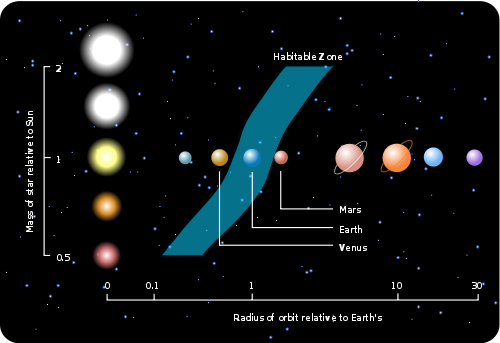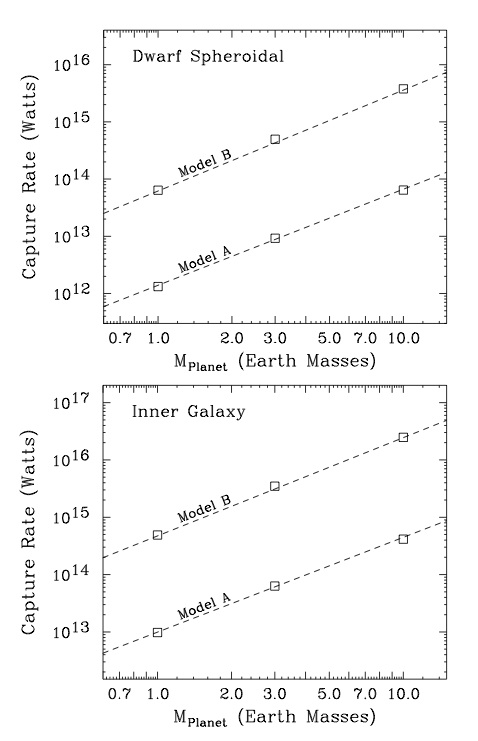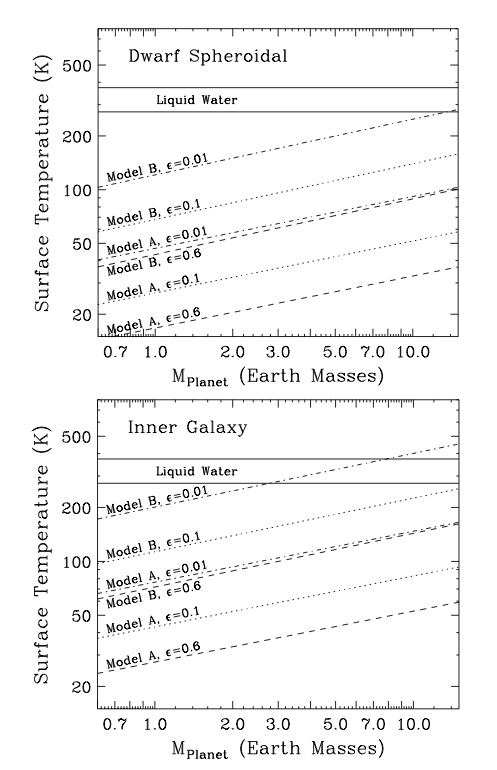# Dark Matter and Planet Habitability

Paper title: Dark Matter and the Habitability of Planets
Authors: D. Hooper and J. H. Steffen
First Author’s Affiliation: Center for Particle Astrophysics, Fermilab; Department of Astronomy and Astrophysics, University of Chicago

This eye-catching theory paper asks an elegant but simple question: when dark matter is gravitationaly captured by a planet, can the energy released when it annihalates provide enough heat to make the planet habitable?

Background: Planet Habitability

Planet habitability is generally defined as being able to support liquid water on the surface. In the regime of Earthlike planets, this maps into having surface temperatures in the range of 273-373K.

If we assume that the planet is in thermal equilibrium and that it radiates as a blackbody, we can determine the surface temperature by equating the energy flux$sigma epsilon T_{eff}^{4}$ (Stefan-Boltzmann Law) radiated by the planet surface with the energy flux recieved by the surface. In this expression,$sigma$ is a constant and$T_{eff}$ is the effective blackbody temperature of the planet. The authors account for the greenhouse effect with$epsilon$, the emissivity (~.6 for the Earth); only some fraction of the radiated energy will ultimately escape, and the rest will be retained by the planet’s atmosphere. Therefore,

Equation 1:$P_{tot} = sigma epsilon T_{eff}^{4}$

Sources of inward flux include stellar irradiation, radiogenic decay of unstable isotopes in the planet core, and tidal heating in the case of bodies with large partner bodies. For bodies in the solar system irradiation generally strongly dominates over other sources. Consequently, we often approximate

Equation 2:$P_{tot} = sigma epsilon T_{eff}^{4} approx P_{star} = L_{star}frac{R_{planet}^{2}}{4D^2}(1-a)$,

Where$a$ is the planet’s albedo (what fraction of radiation it reflects back to space) and$D$ is the star-planet distance. By plugging this expression in for$P_{tot}$ in equation 1 above and solving for D for the boundary cases of T=273 and 373 K, we may determine the spatial extent of the habitable zone for a given star. The classic paper of (Kastings, Whitemire and Reynolds 1993) performs a slightly more sophisticated version of this calculation to obtain the habitable zone for different stellar classes.Figure 1: Illustration of the classical liquid water habitable zone for different stellar types. From http://astronomy.nmsu.edu/tharriso/ast105/Exoplanets.html.

Heating From Dark Matter
This paper considers another energy source: dark matter, specifically the dark matter candidates known as Weakly Interacting Massive Particles (WIMPs). These particles are predicted to interact with nuclei. In theory, they can be elastically scattered such that they lose momentum to these nuclei and can be gravitationally captured by massive objects, like planets. This capture rate increases with the mass of the capturing body, the density of the medium of scattering and the density of WIMPs, and falls off with increasing relative speed of the WIMPs.

Once bound, the authors assert that the particles will eventually annihilate (the annihilation mechanism is not specified), releasing energy into the environment. The annihalation end products are assumed to be capable of interacting with baryonic matter. This should provide an internal energy source similar to radiogenic heating that can then convect and conduct to the surface. Sufficiently large dark matter heating could extend the habitable zone outwards. It could even render habitable rogue planets, which are worlds that have been ejected from their solar system into interstellar space.

How much heating should we expect? To obtain quantitative estimates, the authors make a few more assumptions. First, they assume that the dark matter capture and annihilation processes will eventually reach equilibrium: as the concentration of captured dark matter increases, the annihilation rate rises, until the rates of annihilation and capture are equal. Secondly, they assume a planet with a core composition and structure similar to Earth’s. Finally, they consider two different WIMP models. Model A is based on typical WIMP models from supersymmetry and other theoretical considerations; it has a mass-energy of 300 GeV and a spin-independent cross-section of$8 times 10^{-44}$ cm^2. Model B is motivated by signals reported by dark matter detection experiments (CoGeNT, DAMA/LIBRA) in 2010. It has a mass of 7 GeV and a cross-section of$2 times 10^{-40}$ cm^2. It should be mentioned that these results are quite controversial, and represent an optimistic scenari.

The authors then proceed to compute the capture (and thus heating) rate as a function of planet mass for Earthlike planets in the solar neighborhood. They explicitly compute the capture rate for 1, 3 and 10 Earth masses, and then construct a linear interpretation in log-log space (a power law). They assume a dark matter local density of 0.4 GeV/cm^3 (observationally motivated), a relative velocity of 250 km/s (velocity of sun with respect to the LSR), and a velocity dispersion of 250 km/s. They do not provide the formula used to perform this calculation. Their results are plotted in Figure 2 below:Figure 2: (Hooper and Steffen 2010) Figure 1, plotting mass-energy of captured WIMPs versus planet mass. The capture energy is explicitly computed for 1, 3 and 10 Earth masses and interpolated for the rest. More massive planets capture more mass-energy and are thus better heated. Model B provides higher heating rates courtesy of its much higher cross-section.

Effect of Planet Location
The heating rates for both model A and model B fall far short by well over 7 orders of magnitude from what is required to sustain a habitable Earthlike world ($epsilon approx .6$). However, this computation was performed for the solar neighborhood, with relatively high relative velocity dispersions and low dark matter concentration. What about more favorable locations? The authors consider two of these: dwarf galaxies and the center of the Milky Way, both of which should have lower
velocity dispersions and higher dark matter densities. The authors consider the case of an Earthlike planet located 10 pc from the center of a dwarf galaxy with local dark matter density of 150 GeV/cm^3 and velocity dispersion of 10 km/s. This is at the most optimistic end of what is justified by observations. The authors also consider the case of an Earthlike planet 10 pc from the center of the Milky Way, with dark matter density of 825 GeV/cm^3, estimated using a modified NFW profile, and velocity dispersion of 8.6 km/s, estimated using scaling relations. Repeating the analysis performed for Figure 2 for each of these cases, the authors obtain Figure 3 below.Figure 3: (Hooper and Steffen 2010) Figure 2, plotting mass-energy of captured WIMPs versus planet mass for the centers of a dwarf galaxy and the Milky Way. Note the ~7 orders of magnitude improvement in energy delivery relative to the solar neighborhood. The Milky Way provides more dark matter heating.

The authors then use Equation 1 above to compute expected surface temperature as a function of planet mass under the assumption that dark matter heating is the sole input power source. They compute these curves for a variety of different emissivities$epsilon$. The results are shown in Figure 4. For comparison purposes, Earth has$epsilon_{Earth} approx 0.6$, while Venus, with its strong greenhouse effect that retains much of its radiant energy, has$epsilon_{Venus} approx 0.004$.Figure 4: (Hooper and Steffen 2010) Figure 3, showing expected mean surface temperature for purely dark-matter heated planets for different emissivities and WIMP models as a function of mass. The liquid water habitable zone is marked on the plot.

The authors interpret these results to indicate that dark-matter heated habitable planets are plausible. The$epsilon=.01$ model with type B WIMPs does indeed achieve the habitable zone in the galactic center. However, none of the other models succeed in doing so, rendering this result strongly dependent on assumptions as to the nature of dark matter. Low emissivities are also required to achieve habitability. Given the specificity of the assumptions required, we should approach the concept of habitable rogue planets with a great deal of caution.

Even if dark matter heating alone is incapable of rendering planets habitable, one can imagine that it might provide energy inputs on the scale of radiogenic heating on planets near galactic centers ($approx 3times 10^{13}$ Watts on Earth). As such, it might still be relevant for climate considerations. We can also imagine that it might abet volcanism and plate tectonics, both of which are important for the development of life as we know it.

Conclusions
The authors explore an interesting approach to heating planets: annihilation from captured dark matter particles, in particular WIMPs. They examine two separate models of WIMPs motivated by theoretical and experimental considerations, and compute the annihilation rate in planets of different mass under assumptions of annihilation/capture equilibrium. While dark matter heating alone is incapable of rendering a planet habitable in the vicinity of the solar system, low-emissivity super-Earths in the neighborhoods of the centers of dwarf galaxies and the Milky Way may be able to sustain liquid water thanks to this mechanism. This result should be approached with caution as it is strongly dependent on the precise nature of as-yet-undetected dark matter. We might envision cloud-shrouded worlds wandering interstellar space with life thriving on dark matter annihilation! Even if incapable of rendering planets habitable on its own, dark matter heating might abet volcanism and plate tectonics, helping create an environment more conducive to the emergence of life as we know it.